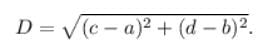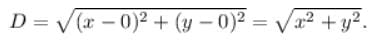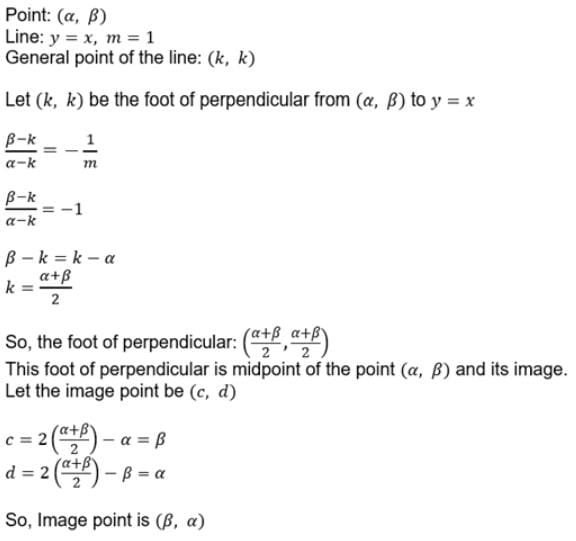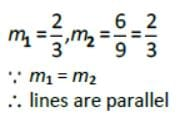JEE  >  Test: Straight Lines- 1

# Test: Straight Lines- 1

Test Description

## 25 Questions MCQ Test Mathematics (Maths) Class 11 | Test: Straight Lines- 1

Test: Straight Lines- 1 for JEE 2023 is part of Mathematics (Maths) Class 11 preparation. The Test: Straight Lines- 1 questions and answers have been prepared according to the JEE exam syllabus.The Test: Straight Lines- 1 MCQs are made for JEE 2023 Exam. Find important definitions, questions, notes, meanings, examples, exercises, MCQs and online tests for Test: Straight Lines- 1 below.
Solutions of Test: Straight Lines- 1 questions in English are available as part of our Mathematics (Maths) Class 11 for JEE & Test: Straight Lines- 1 solutions in Hindi for Mathematics (Maths) Class 11 course. Download more important topics, notes, lectures and mock test series for JEE Exam by signing up for free. Attempt Test: Straight Lines- 1 | 25 questions in 25 minutes | Mock test for JEE preparation | Free important questions MCQ to study Mathematics (Maths) Class 11 for JEE Exam | Download free PDF with solutions
 1 Crore+ students have signed up on EduRev. Have you?
Test: Straight Lines- 1 - Question 1

### The point which divides the joint of (1, 2) and (3,4) externally in the ratio 1 : 1.

Detailed Solution for Test: Straight Lines- 1 - Question 1

x1 = (1*3 - 1*1)/(1-1)
y1 = (1*4 - 1*2)/(1-1)
Hence the denominator becomes zero, here we can say that any point cannot be divided in the ratio of 1:1 externally.

Test: Straight Lines- 1 - Question 2

### The distance of the point (x, y) from the origin is

Detailed Solution for Test: Straight Lines- 1 - Question 2

Step-by-step explanation:  We are given to find the distance of the point (x, y) from the origin.

We know that the co-ordinates of the origin are (0, 0).

And, the distance between any two points (a, b) and (c, d) is given by the following distance formula:Therefore, the distance between the point (x, y) and the origin will beTest: Straight Lines- 1 - Question 3

### The distance of the point (x , y) from Y axis is

Detailed Solution for Test: Straight Lines- 1 - Question 3

Distance is a metric, a bifunction d, which is always non-negative, along with being symmetric, satisfies the triangle inequality, and the identity of indiscernibles (i.e., d(x,y)=0⟺x=y)
d(x,y)=0⟺x=y). The “nearest” distance from a point (x1,y1) to the y-axis in 2-space is along the line y=y1. (orthogonal to the y-axis). This line interests the y-axis exactly at the point (0,y1). Using the Euclidean distance metric on R2, one obtains:
d((x,y),(0,y))= √(x−0)2+(y−y)2
= |x|

Test: Straight Lines- 1 - Question 4

Slope of a line is not defined if the line is

Test: Straight Lines- 1 - Question 5

The distance of the point (α,β) from X axis is

Test: Straight Lines- 1 - Question 6

The line through the points (a , b) and (- a, - b) passes through the point

Detailed Solution for Test: Straight Lines- 1 - Question 6

Slope of line passing through (a,b) and (−a,−b) is given by (b+b)/(a+a) = b/a
So equation of line passing is given by (using slope point form)
y−b = b/a(x−a)
⇒ ay − ab = bx − ab
⇒ ay = bx
Clearly the point (a2,ab) lie on the above line

Test: Straight Lines- 1 - Question 7

Projection (the foot of perpendicular) from (x , y) on the x – axis is

Detailed Solution for Test: Straight Lines- 1 - Question 7

Slope of a line is not defined if the line is parallel to x axis as all points on the line have same x coordinate.

Test: Straight Lines- 1 - Question 8

The image of the point (α,β) is the line x + y = 0

Test: Straight Lines- 1 - Question 9

The distance between the lines 5x – 12y + 65 = 0 and 5x – 12y – 39 = 0

Detailed Solution for Test: Straight Lines- 1 - Question 9

5x − 12y + 65 = 0, 5x − 12y − 39 = 0
Since, these lines are parallel .
a = 5,  b = -12,  c1 = 65    c2 = -39
So, d = |c1 − c2|/[a2 + b2]1/2
⇒ d = 104/13
= 8

Test: Straight Lines- 1 - Question 10

The image of the point (α,β) is the line x = y is

Detailed Solution for Test: Straight Lines- 1 - Question 10Test: Straight Lines- 1 - Question 11

The equation y−y1 = m (x−x1), m ∈ R, represents all lines through the point (x1,y1) except the line

Test: Straight Lines- 1 - Question 12

The distance between the parallel lines 3x + 4y + 13 = 0 and 3x + 4y – 13 = 0 is

Detailed Solution for Test: Straight Lines- 1 - Question 12

Given parallel lines3x +4y+13=0
perp length from origin P1= 13/√(3)2 +(4)2
=13/5
and another line 3x+4y-13=0 P2= -13/√(3)2+(4)2
=-13/5
Now distance b/w parallel lines
D=P1-P2
=13/5+13/5
=26/5 units

Test: Straight Lines- 1 - Question 13

Slope of any line parallel yo X axis is

Test: Straight Lines- 1 - Question 14

The straight lines x + y = 0 , 3x + y – 4 = 0 , x + 3y – 4 = 0 form a triangle which is

Detailed Solution for Test: Straight Lines- 1 - Question 14

Explanation:- x+y=0 .....  (i)
3x+y−4=0 ..... (ii)
x+3y−4=0 ....... (iii)
Solving lines (i) and (ii), we get
−x=−3x+4
⟹ x=2, y=−2
∴(i) and (ii) intersect at A=(2,−2)
Solving lines (ii) and (iii), we get
−3x+4 = (4−x)/3
⟹ −9x+12 = 4−x
⟹x=1, y=1
∴(ii) and (iii) intersect at B=(1,1)
Solving lines (i) and (iii), we get
-x = (4−x)/3
⟹ −3x = 4−x
⟹ x=−2, y=2
So, AB,BC,AC form a triangle ABC
Now, AB = [(1−2)2 + (1+2)2]1/2 = (10)1/2
BC = [(−2−1)2 + (2−1)2]1/2 = (10)1/2
AC = [(−2−2)2 + (2+2)2]1/2 = (32)1/2
​∴ AB=BC
Since, two sides of a triangle are equal then the triangle formed by A,B,C is isosceles triangle.

Test: Straight Lines- 1 - Question 15

The lines 2x – 3y = 5 and 6x – 9y – 7 = 0 are

Detailed Solution for Test: Straight Lines- 1 - Question 15Test: Straight Lines- 1 - Question 16

The equation of the line which passes through the point (1 , - 2) and cuts off equal intercepts from the axis is

Test: Straight Lines- 1 - Question 17

If a line is drawn through the origin and parallel to the line x – 2y + 5 = 0 , its equation is

Test: Straight Lines- 1 - Question 18

The line passing through (1, 1) and parallel to the line 2x – 3y + 5 = 0 is

Detailed Solution for Test: Straight Lines- 1 - Question 18

Correct option (A) 2x - 3y + 1 = 0

Explanation:

Let the required line is 2x - 3y + k = 0

it passes through (1, 1)

:. 2 - 3 + k = 0

k = 1

:. required line is 2x - 3y + 1 = 0

Test: Straight Lines- 1 - Question 19

The equation of the line which passes through the point (2 , - 3) and cuts off equal intercepts from the axis is

Test: Straight Lines- 1 - Question 20

The vertex A of a triangle ABC is the point (-2, 3) whereas the line perpendicular to the sides AB and AC are x – y – 4 = 0 and 2x – y – 5 = 0 respectively. The right bisectors of sides meet at P(3/2 , 5/2) . Then the equation of the median of side BC is

Detailed Solution for Test: Straight Lines- 1 - Question 20

Given vertex of ΔABC A(−2,3)
Equation of side AB perpendiuclar to x−y−4=0 is
AB:x+y−λ=0
Side AB passes through point A(−2,3)
−2+3−λ=0
λ=1
Hence AB:x+y−1=0−−−−(1)
Equation of side AC perpendiuclar to 2x−y−5=0 is
AC:x+2y−λ=0
Side AC passes through point A(−2,3)
−2+6−λ=0
λ=4
Hence AC:x+2y−4=0−−−−(2)
The right bisectors of all sides are meeting at point P(3/2 , 5/2)
Right bisector from point B on side AC is perpendicular
Hence Equation of right bisector perpendicular to x+2y−4=0 is
2x−y−λ=0
Here right bisector is passing through point P (2 × 3/2 − 5/2​)λ = 0
=> 6/2 − 5/2 = λ
λ=1/2
Equation of right bisector BD be
2x−y−1/2 =0
4x−2y−1=0−−−−(3)
Here On solving equation of AB and BD we get point of intersection i.e. point B
4(1−y)−2y−1=0
4−4y−2y−1=0
−6y=−3
y = 1/2
​x = 1 − y = 1− 1/2 = 1/2
Point B(1/2,1/2)
Right bisector from point A on side BC is passing through point P
Hence equation of AD from point A and P
y−3 = {[5/2−3](x+2)/(3/2+2)}
y−3 = −1/7(x+2)
Hence slope of side BC perpendicular to AD is −1/7mBC = −1
mBC = 7
Equation of side BC from point B(1/2,1/2) with slope mBC=7
y − 1/2 = 7(x−1/2)
2y−1=14x−7
14x−2y−6=0

Test: Straight Lines- 1 - Question 21

The distance between the lines 4x + 3y = 11 and 8x + 6y = 15 is ?

Detailed Solution for Test: Straight Lines- 1 - Question 21

Given lines are 4x + 3y = 11 and 4x + 3y = 15/2
Distance between two parallel lines = |c₁ - c₂ / √a² + b²|
= | 11 - 15/2/ √16 + 9 |
= | 7 / 2 × 5 |
= 7/10

Test: Straight Lines- 1 - Question 22

The line which passes through the point (0 , 1) and perpendicular to the line x – 2y + 11 = 0 is

Test: Straight Lines- 1 - Question 23

Thee perpendicular distance of the origin from the line 3x +4y + 1 = 0 is

Test: Straight Lines- 1 - Question 24

The acute angle between the lines x – y = 0 and y = 0 is

Detailed Solution for Test: Straight Lines- 1 - Question 24

Line y = 0  is X-axis slope of this line = 0 = m1
line x-y = 0 is y = x which is passes through (0,0)  slope of this line = 45 = m2
tanФ = (m2-m1)/(1-m1m2) = (1-0)/(1-1*0)
= 1/1 = 1
tanФ =1
Ф = 45

Test: Straight Lines- 1 - Question 25

The vertices of a triangle are (0 , 3) , (- 3 , 0) and (3 , 0). The orthocenter of the triangle is

## Mathematics (Maths) Class 11

156 videos|176 docs|132 tests
 Use Code STAYHOME200 and get INR 200 additional OFF Use Coupon Code
Information about Test: Straight Lines- 1 Page
In this test you can find the Exam questions for Test: Straight Lines- 1 solved & explained in the simplest way possible. Besides giving Questions and answers for Test: Straight Lines- 1, EduRev gives you an ample number of Online tests for practice

## Mathematics (Maths) Class 11

156 videos|176 docs|132 tests